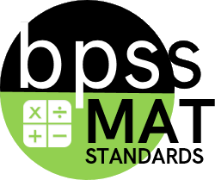(OA) Operations and Algebraic Thinking Categories All categories Not categorized (G) Geometry (MD) Measurement and Data (NBT) Number and Operations in Base Ten (OA) Operations and Algebraic Thinking Prioritized

# Operations and Algebraic Thinking

## Narrative for the (OA) Operations and Algebraic Thinking

Algebraic thinking is about generalizing arithmetic operations and operating on unknown quantities.  It involves recognizing and analysing patterns and developing generalizations about these patterns.  In algebra, symbols can be used to represent generalizations. Operations and Algebraic Thinking deals with the basic operations - addition, subtraction, multiplication, and division - the relationships they model, the kinds of problems they can be used to solve, as well as, their mathematical properties and relationships.

Grade 2 students build upon their work in Grade 1 with operations and algebraic thinking in two major ways. They represent and solve situational problems which involve addition and subtraction within 100 rather than within 20, and they represent and solve two-step situational problems in which the result is unknown, the change is unknown, and the start is unknown. (20 + 15 = ___; 20 + ___ = 46; ___ + 14=26)

## Calculation Method for Domains

Domains are larger groups of related standards. The Domain Grade is a calculation of all the related standards. Click on the standard name below each Domain to access the learning targets and rubrics/ proficiency scales for individual standards within the domain.

#### MAT-02.OA.012nd Grade (MAT) Targeted Standard
(OA) Operations and Algebraic Thinking
Cluster: Use place value understanding and properties of operations to add and subtract.

#### MAT-02.OA.022nd Grade (MAT) Targeted Standard
(OA) Operations and Algebraic Thinking
Cluster: Add and subtract within 20.

#### MAT-02.OA.02.a

 MAT-02 Targeted Standards(OA) Domain: Operations and Algebraic ThinkingCluster: Add and subtract within 20. MAT-02.OA.02.a Fluently add and subtract within 20 using mental strategies. By end of Grade 2, know from memory all sums of two one-digit numbers. Know from memory: The ability to compute math facts with automaticity (3-5 seconds) and confidence (ND).

## Resources

### Vocabulary

• sum
• know from memory
• plus
• equals

#### MAT-02.OA.02.b

 MAT-02 Targeted Standards(OA) Domain: Operations and Algebraic ThinkingCluster: Add and subtract within 20. MAT-02.OA.02.b Fluently add and subtract within 20 using mental strategies. By end of Grade 2, know from memory all sums of two one-digit numbers. Know from memory: The ability to compute math facts with automaticity (3-5 seconds) and confidence (ND).

## Student Learning Targets:

### Knowledge Targets

• I can answer subtraction facts to 20 quickly (3-5 seconds).

## Resources

### Vocabulary

• subtraction facts
• difference
• know from memory
• minus
• subtract
• equals

#### MAT-02.OA.03

Under Development

 MAT-02 Targeted Standards(OA) Domain: Operations and Algebraic ThinkingCluster: Work with equal groups of objects to gain foundations for multiplication. MAT-02.OA.03 Determine whether a group of objects (up to 20) has an odd or even number of members, e.g., by pairing objects or counting them by 2s; write an equation to express an even number as a sum of two equal addends.

• I can

• I can

• I can

• I can

## Proficiency (Rubric) Scale

 Score Description Sample Activity 4.0 Student is able to - 3.5 In addition to Score 3.0 performance, the student demonstrates in-depth inferences and applications regarding the more complex content with partial success. 3.0 “The Standard.” Student is able to - 2.5 No major errors or emissions regarding 2.0 content and partial knowledge of the 3.0 content. 2.0 Student is able to - 1.5 In addition to 1.0 content, student has partial knowledge of the 2.0 and/or 3.0 content. 1.0 Student is able to - 0.5 Limited or no understanding of the skill id demonstrated.

## Resources

### Vocabulary

• List

#### MAT-02.OA.04

Under Development

 MAT-02 Targeted Standards(OA) Domain: Operations and Algebraic ThinkingCluster: Work with equal groups of objects to gain foundations for multiplication. MAT-02.OA.04 Use addition to find the total number of objects arranged in rectangular arrays with up to 5 rows and up to 5 columns; write an equation to express the total as a sum of equal addends.

• I can

• I can

• I can

• I can

## Proficiency (Rubric) Scale

 Score Description Sample Activity 4.0 Student is able to - 3.5 In addition to Score 3.0 performance, the student demonstrates in-depth inferences and applications regarding the more complex content with partial success. 3.0 “The Standard.” Student is able to - 2.5 No major errors or emissions regarding 2.0 content and partial knowledge of the 3.0 content. 2.0 Student is able to - 1.5 In addition to 1.0 content, student has partial knowledge of the 2.0 and/or 3.0 content. 1.0 Student is able to - 0.5 Limited or no understanding of the skill id demonstrated.